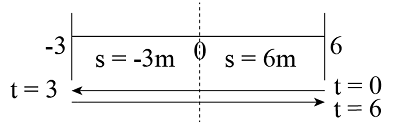# A particle travels along a straight line such that its position is defined by s = (t2 - 6t + 6)...

## Question:

A particle travels along a straight line such that its position is defined by s = (t{eq}^{2} {/eq} - 6t + 6) m. Determine the average velocity, the average speed, and the acceleration of the particle when t = 6 s.

## Velocity:

When an object moves, then the change in the position of the object in a unit time is known as the velocity of the object. It is a vector quantity that is measured in terms of a meter per second.

Given data

• Position of the particle: {eq}S = \left( {{t^2} - 6t + 6} \right) {/eq}.
• Time of travel of the particle: {eq}t = 6\;{\rm{s}} {/eq}.

Differentiate the equation of posiont of the particle with respect to time.

{eq}\begin{align*} \dfrac{{dS}}{{dt}} &= \dfrac{d}{{dt}}\left( {{t^2} - 6t + 6} \right)\\ V &= 2t - 6 \end{align*} {/eq}

Here, {eq}V {/eq} is the velocity of particle.

Calculate the time of rest of the particle, take {eq}V\; {/eq}equal to zero.

{eq}\begin{align*} V &= 0\\ 2t - 6 &= 0\\ t &= 3\;{\rm{s}} \end{align*} {/eq}

Calculate the position at {eq}t = 0\;{\rm{s}} {/eq}.

{eq}\begin{align*} {S_0} &= {0^2} - \left( {6 \times 0} \right)\;{\rm{m}} + 6\;{\rm{m}}\\ {{\rm{S}}_0} &= 6\;{\rm{m}} \end{align*} {/eq}

at {eq}t = 3\;{\rm{s}} {/eq}.

{eq}\begin{align*} {{\rm{S}}_3} &= {3^2} - 6 \times 3 + 6\\ {S_3} &= - 3\;{\rm{m}} \end{align*} {/eq}

at {eq}t = 6\;{\rm{s}} {/eq}.

{eq}\begin{align*} {{\rm{S}}_6} &= {6^2} - 6 \times 6 + 6\\ {S_6} &= 6\;{\rm{m}} \end{align*} {/eq}(a)

The expression for the average velocity of the particle is as follows.

{eq}{V_{avg}} = \dfrac{{\Delta S}}{{\Delta t}} {/eq}

Here, {eq}\Delta S {/eq} is change in initial position to final position.

{eq}\begin{array} \Delta S &= {S_0} - {S_6}\\ \Delta S &= 6 - 6\\ \Delta S &= 0\;{\rm{m}} \end{array} {/eq}

The value of {eq}\Delta t = 6\;{\rm{s}} {/eq}.

Substitute all the values in above expressions.

{eq}\begin{align*} {V_{avg}} &= \dfrac{0}{6}\\ {V_{avg}} &= 0\;{{\rm{m}} {\left/ {\vphantom {{\rm{m}} {\rm{s}}}} \right. } {\rm{s}}} \end{align*} {/eq}

Thus, the value of average velocity is {eq}0\;{{\rm{m}} {\left/ {\vphantom {{\rm{m}} {\rm{s}}}} \right. } {\rm{s}}}. {/eq}

(b)

The expression for the average speed of the particle is as follows.

{eq}{v_{avg}} = \dfrac{{{S_{total}}}}{{{t_{total}}}} {/eq}

Here, {eq}{v_{avg}} {/eq} is average speed, {eq}{S_{total}} {/eq} is sum of all the travel distance and {eq}{t_{total}}{/eq} is the total time of travel.

{eq}\begin{align*} {S_{total}} &= {S_{0 - 3}} + {S_{3 - 6}}\\ {S_{total}} &= 9\;{\rm{m}} + 9\;{\rm{m}}\\ {S_{total}} &= 18\;{\rm{m}} \end{align*} {/eq}

Substitute all the values in above expressions.

{eq}\begin{align*} {v_{avg}} &= \dfrac{{18\;{\rm{m}}}}{{6\;{\rm{s}}}}\\ {v_{avg}} &= 3\;{{\rm{m}} {\left/ {\vphantom {{\rm{m}} {\rm{s}}}} \right. } {\rm{s}}} \end{align*} {/eq}

Thus, the value of average speed is {eq}{v_{avg}} = 3\;{{\rm{m}} {\left/ {\vphantom {{\rm{m}} {\rm{s}}}} \right. } {\rm{s}}} {/eq}.

(c)

The expression for the acceleration of the particle is as follows.

{eq}a = \dfrac{{dV}}{{dt}} {/eq}

Here, {eq}A {/eq} is the acceleration of the particle.

Substitute the values in above expression.

{eq}\begin{align*} a &= \dfrac{d}{{dt}}\left( {2t - 6} \right)\\ a &= 2\;{{{\rm{m}} {\left/ {\vphantom {{\rm{m}} {\rm{s}}}} \right. } {\rm{s}}}^2} \end{align*} {/eq}

Thus, the value of acceleration of the particle is {eq}2\;{{{\rm{m}} {\left/ {\vphantom {{\rm{m}} {\rm{s}}}} \right. } {\rm{s}}}^2} {/eq}.